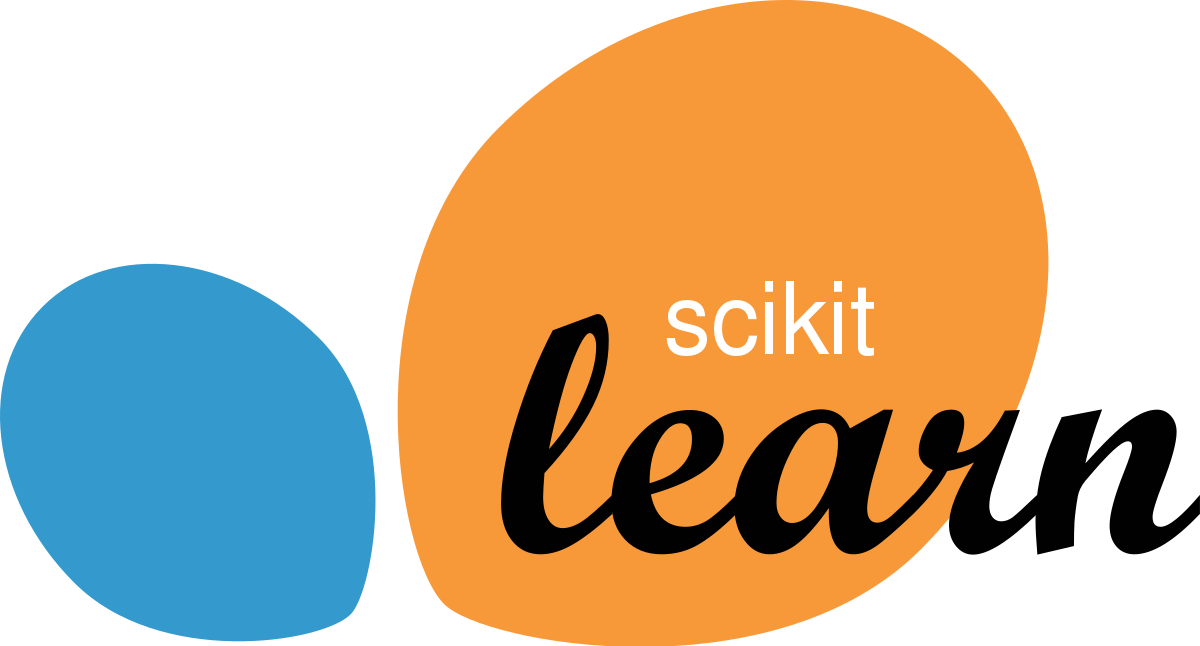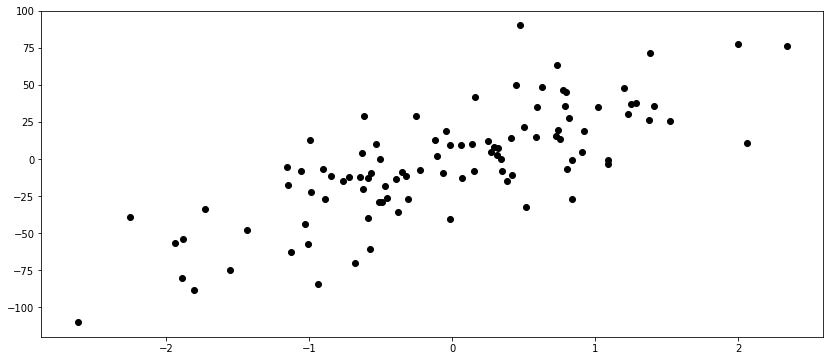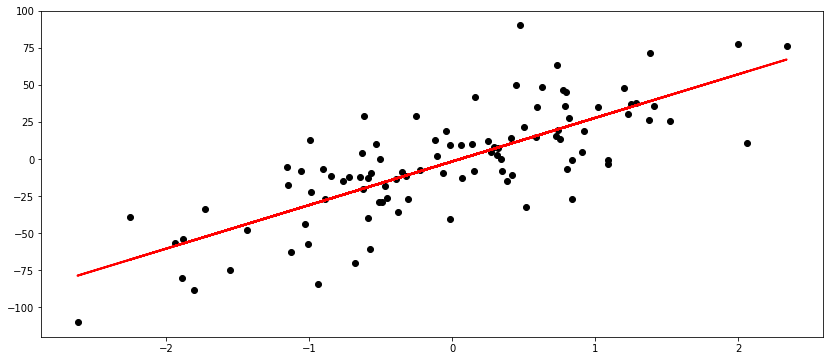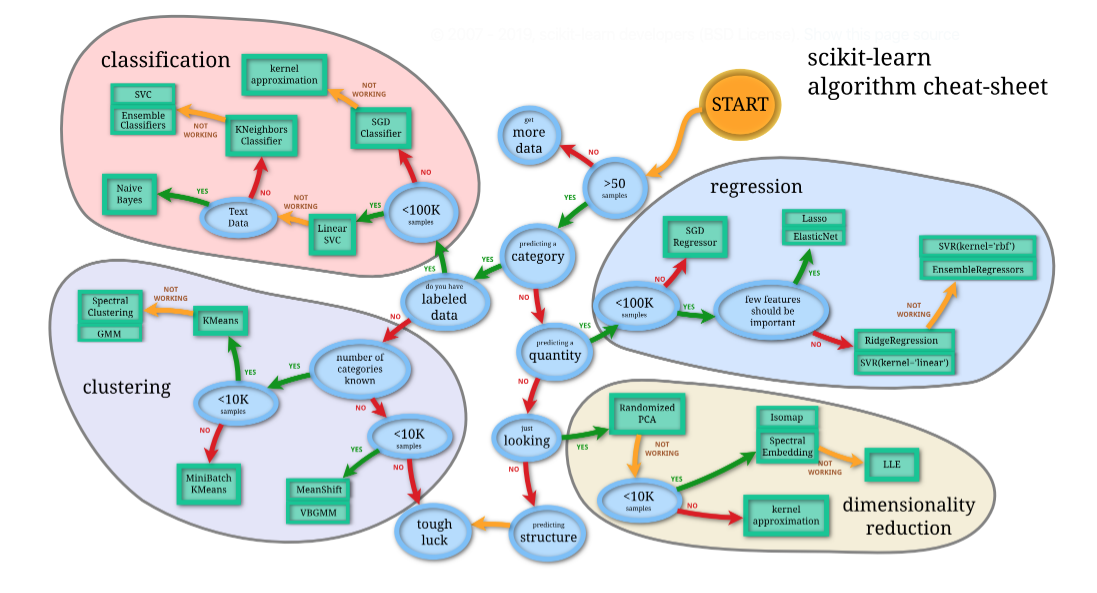# MLF - Scikit-Learn Introduction

Last updated: April 6th, 2020# Scikit-learn introduction¶

This lesson will illustrate some of the main features that scikit-learn provides.## What is scikit-learn?¶Scikit-learn, or sklearn, is an open source machine learning library that supports supervised and unsupervised learning. It also provides various tools for model fitting, data preprocessing, model selection and evaluation, as well as many other utilities:

• Simple and efficient tools for predictive data analysis
• It is accessible to everybody, and reusable in various contexts
• Built on NumPy, SciPy, and matplotlib
• It is open source, commercially usable - BSD license

### Where did it come from?¶

Scikit-learn was initially developed by David Cournapeau as a Google summer of code project in 2007. Later Matthieu Brucher joined the project and started to use it as apart of his thesis work. In 2010 INRIA got involved and the first public release (v0.1 beta) was published in late January 2010.## What can I do with scikit-learn?¶

Scikit-learn offers various important features for machine learning such as classification, regression and clustering algorithms including support vector machines, random forests, gradient boosting, k-means and DBSCAN, and is designed to inter-operate with the python numerical and scientific libraries like NumPy and SciPy.

It provides a large list of tools and algorithms, divided in 6 categories:

• Classification, to identify which category an object belongs to.
• Regression, to predict a continuous-valued attribute associated with an object.
• Clustering, to perform automatic grouping of similar objects into sets.
• Dimensionality reduction, to reduce the number of random variables to consider.
• Model selection, to compare, validate and choose parameters and models.
• Preprocessing, to extract and normalize features.## Estimator Interface¶

All implemented algorithms in scikit-learn, whether preprocessing, supervised learning, or unsupervised learning algorithms, are considered as classes.

These classes are called Estimators in scikit-learn. To apply an algorithm, you first have to instantiate an object of the particular class.

For example, let's see how can we perform a Linear Regression:

In :
from sklearn.linear_model import LinearRegression

model = LinearRegression()

model

Out:
LinearRegression(copy_X=True, fit_intercept=True, n_jobs=None, normalize=False)

The estimator class contains the algorithm, and also stores the model that is learned from data using the algorithm.

You should set any parameters of the model when constructing the model object. These parameters include regularization, complexity control, number of clusters to find, etc.

### Fit method¶

estimator.fit(x_train, [y_train])

All estimators have a fit method, which is used to build the model.

The fit method always requires as its first argument the data X, represented as a NumPy array or a SciPy sparse matrix, where each row represents a single data point. The data X is always assumed to be a NumPy array or SciPy sparse matrix that has continuous (floating-point) entries.

Supervised algorithms also require a y argument, which is a one-dimensional NumPy array containing target values (labels) for regression or classification (i.e., the known output labels or responses).

In :
from sklearn.datasets import make_regression

X, y = make_regression(n_samples=100,
n_features=1,
noise=25)

X.shape, y.shape

Out:
((100, 1), (100,))
In :
X, y

Out:
(array([-1.88059384]), -54.19046754899451)
In :
X, y

Out:
(array([-0.57468891]), -60.873006989033655)
In :
import numpy as np
import matplotlib.pyplot as plt

plt.figure(figsize=(14,6))

plt.scatter(X, y, color='black')

Out:
<matplotlib.collections.PathCollection at 0x7f463e23bc90>In :
model.fit(X, y)

Out:
LinearRegression(copy_X=True, fit_intercept=True, n_jobs=None, normalize=False)

### Predict method¶

estimator.predict(X_test)

There are two main ways to apply a learned model in scikit-learn.

To create a prediction in the form of a new output like $y$, you use the predict method.

To create a new representation of the input data, you use the transform method.

We will cover this transform method in detail on upcoming "Transforming and Preprocessing Data" lesson.

In :
y_pred = model.predict(X)

y_pred

Out:
array([-56.87676976, -18.52929226,  20.55085273,  36.00576867,
-16.79077584,  10.46661021,  43.06181834,  -9.19914619,
7.50068815, -28.17275135,  -4.78569563, -19.87913082,
3.11201818, -19.04284791,  34.3639909 , -11.26093156,
30.39838899, -21.6087578 ,   6.99448875,   8.46440758,
-54.6278653 ,  23.04327734,   8.37843557, -30.89068465,
11.45474552,  57.00257289,  67.04104006,  16.68623123,
-22.8243619 , -32.82312605,  -2.05701209,  21.50552102,
-67.85484689,   9.51203944,  19.84176227, -35.51805483,
-11.88318712,  22.36454305, -12.74686122, -26.53429866,
10.66272963,   6.30367449,  38.98491447, -35.36625399,
-78.65359723, -43.76530854, -17.37214446, -52.40281301,
58.95206954,  39.82200653, -57.25181985,  21.06883991,
15.70558524,  21.84060633, -20.15999166,  38.75073323,
-24.16009201,  -8.34386849, -20.09420705,  13.07291612,
-15.12620734,   0.32830955, -13.19651863,  25.38974477,
-18.40189473,   0.10031264, -31.2514417 ,  12.1980733 ,
2.32137539, -16.40896339,  21.62167005,  15.56523096,
-15.51257069, -10.75299779,  25.0997835 , -29.31438776,
-2.92701072,  -2.0413947 ,  22.99867099, -16.07028565,
-58.61375385,  35.16291928, -18.97500877, -20.53010914,
-47.23183557,  30.29081384,  -3.52549502,  28.29994913,
20.10214564,  13.53788682, -27.77803329,  -5.23103169,
7.66950604, -30.71217006,   2.87496998,  33.73300633,
-34.86716435,   5.61214258, -31.97065006,  19.75486428])
In :
X, y, y_pred

Out:
(array([-1.88059384]), -54.19046754899451, -56.87676976195995)
In :
X, y, y_pred

Out:
(array([-0.57468891]), -60.873006989033655, -18.529292263794122)
In :
plt.figure(figsize=(14,6))

plt.scatter(X, y, color='black')

plt.plot(X, y_pred, color='red', linewidth=2)

Out:
[<matplotlib.lines.Line2D at 0x7f463df83f90>]### Score method¶

estimator.score(X_test, y_test)

Additionally, all supervised models have a score method that allows an evaluation of the model.

In :
model.score(X, y)

Out:
0.5927049380851392## Choosing the right estimator¶

Often the hardest part of solving a machine learning problem can be finding the right estimator for the job.

Different estimators are better suited for different types of data and different problems.

The flowchart below is designed to give users a bit of a rough guide on how to approach problems in regard to which estimators you should try on your data.